# 2nd PUC Physics Question Bank Chapter 8 Electromagnetic Waves

## Karnataka 2nd PUC Physics Question Bank Chapter 8 Electromagnetic Waves

### 2nd PUC Physics Electromagnetic Waves NCERT Text Book Questions and Answers

Question 1.
Figure 8.6 shows a capacitor made of two circular plates each of radius 12 cm, and separated by 5.0 cm. The capacitor is being charged by an external source (not shown in the figure). The charging current is constant and equal to 0.15A.
(a) Calculate the capacitance and the rate of charge of potential difference between the plates.
(b) Obtain the displacement current across the plates.
(c) Is Kirchhoff’s first rule (junction rule) valid at each plate of the capacitor? Explain.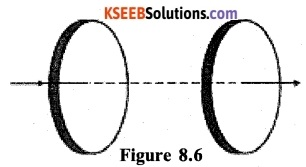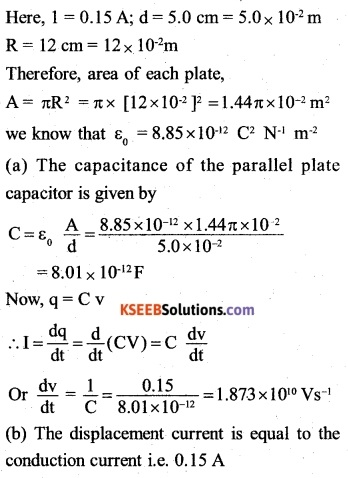(c) Yes, Kirchhoff’s first law will be valid at each plate of the capacitor, provided the electric current means the sum of the conduction and the displacement currents.

Question 2.
A parallel plate capacitor (Fig. 8.7) made of circular plates each of radius R = 6.0 cm has a capacitance C = 100 pF. The capacitor is connected to a 230 Y ac supply with an (angular) frequency of 300 rad s1.
(a) What is the rms value of the conduction current?
(b) Is the conduction current equal to the displacement current?
(c) Determine the amplitude of B at a point 0 cm from the axis between the plates.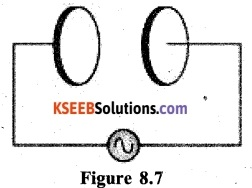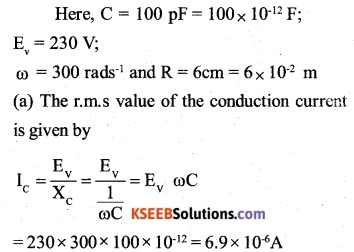(b) Yes the conduction current and the displacement current will be equal. It is true, even if the conduction current is oscillating.

(c) Let us first derive expression for the amplitude of magnetic field at a distance x from the axis of the capacitor. To do so, place a circular loop of radius r between the two plates of the capacitor with its face parallel to the plates and its centre on the axis of the plates.

According to modified Ampere’s circuital law,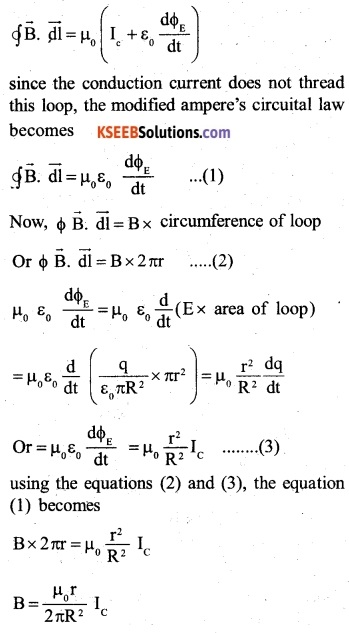The amplitude of magnetic field at a distance r from the axis of the plates is then, given by.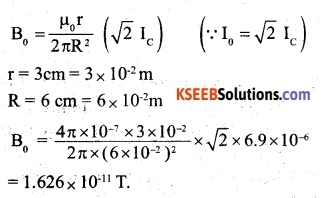Question 3.
What physical quantity is the same for X- rays of wavelength 10-1 m, red light of wavelength 6800 A and radio waves of wavelength 500m?
Both X-rays and red light are parts of the electromagnetic spectrum. They travel with the same speed in Vaccum

Question 4.
A plane electromagnetic wave travels in vacuum along z-direction. What can you say about the directions of its electric and magnetic field vectors? If the frequency of the wave is 30 MHz, what is its wavelength?
The electric and magnetic field vectors are in X Y – plane,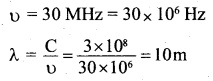Question 5.
A radio can tune in to any station in the 7.5 MHz to 12 MHz band. What is the corresponding wavelength band?
Corresponding to v=7.5 MHz
=7.5×106Hz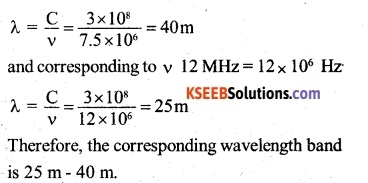Question 6.
A charged particle oscillates about its mean equilibrium position with a frequency of 109 What is the frequency of the electromagnetic waves produced by the oscillator?
The frequency of the electromagnetic waves produced is same as that of the oscillating charged particle. Hence the frequency of the electro­magnetic waves produced is, υ= 109 Hz.Question 7.
The amplitude of the magnetic field part of a harmonic electromagnetic wave in vacuum is Bo = 510 nT. What is the amplitude of the electric field part of the wave
B0 = 510 nT = 510 x 10-9T
The amplitude of the electric field part of the wave,
E0 =CB0 =3 x l08 x 510 x l0-9 =153NC-4

Question 8.
Suppose that the electric field amplitude of an electromagnetic wave is E0 = 120 N/ C and that its frequency is v = 50.0 MHz.
(a) Determine, B0,ω, k, and λ,
(b) Find expressions for E and B.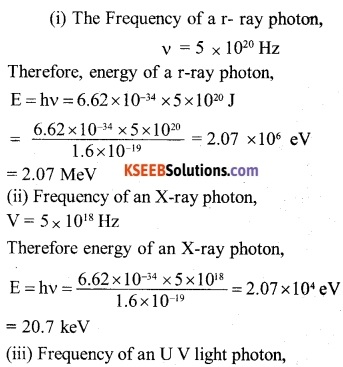Question 9.
The terminology of different parts of the electromagnetic spectrum is given in the text. Use the formula E = hv (for energy of a quantum of radiation: photon) and obtain the photon energy in units of eV for different parts of the electromagnetic spectrum. In what way are the different scales of photon energies that you obtain related to the sources of electromagnetic radiation?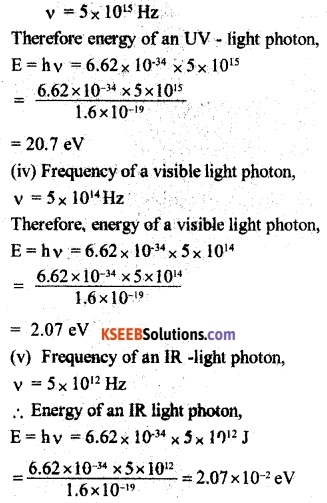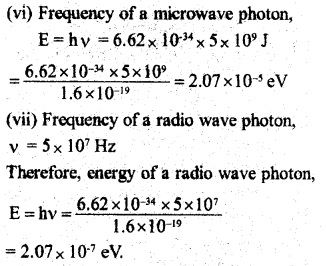Question 10.
In a plane electromagnetic wave, the electric field oscillates sinusoidally at a frequency of 2.0 x 1010 Hz and amplitude 48 V m-1.
(a) What is the wavelength of the wave?
(b) What is the amplitude of the oscillating magnetic field?
(c) Show that the average energy density of the E field equals the average energy density of the B field, [c = 3 x 108 ms-1]
u =2 x 1010Hz
E0 = 48 Vm4 C
= 3 x l08ms-1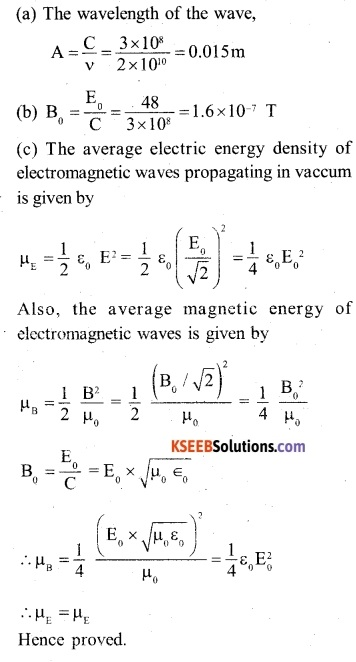Question 11.
Suppose that the electric field part of an electromagnetic wave in vacuum is
E= {(3.1 N/C) cos [(1.8 rad/m) y + (5.4 x 106 rad/s)t]} $$\hat { i }$$

• What is the direction of propagation?
• What is the wavelength λ?
• What is the frequency V?
• What is the amplitude of the magnetic field part of the wave?
• Write an expression for the magnetic field part of the wave.

The electric field part of the electromagnetic wave is given by,
$$\vec { E }$$ = (3.1 NC-1 ) cos [(1,8 rad m-1) y + (5.4x 106 rad s1) t]$$\hat { i }$$ it follows that
E0 = 3.1 NC-1
(a) Since the argument of since in the expression for electric field is of type (ky +cot), the direction of propagation of electro magnetic wave is along negative Y-axis.

(b) The wavelength of the wave,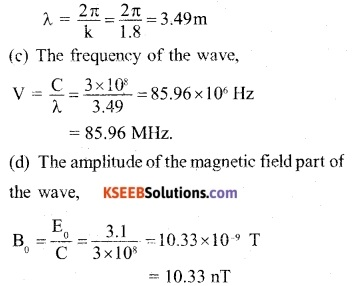(e) The electric field and the direction of propagation of the e.m. wave are along negative X-axis and negative Y-axis respectively. Therefore, the magnetic field is along negative Z-axis and expression for it is given by
B = (10.33nT) cos [(1.8 rad m-1) y + (5.4 x 106rad s-1)t] $$\hat { k }$$Question 12.
About 5% of the power of a 100 W light bulb is converted to visible radiation. What is the average intensity of visible radiation
(a) At a distance of lm from the bulb?
(b) At a distance of 10 m? Assume that the radiation is emitted isotropically and neglect reflection.
P = 100 W
n = 5%
Since the efficiency of the bulb is 5%, the effective power of the bulb.
$${ P }_{ eff }=p\times n=100\times \frac { 5 }{ 100 } =5w$$
At a distance, ‘r’ the radiation coming from the bulb is distributed over the surface of a sphere of radius r i.e. over an area.
A = 4πr2
Therefore, intensity of a light at a distance of r,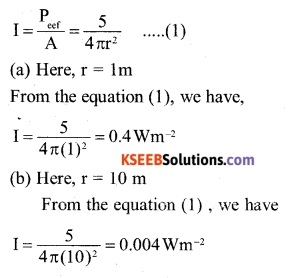Question 13.
Use the formula λmT =29 cmK to obtain the characteristic temperature ranges for different parts of the electromagnetic spectrum. What do the numbers that you obtain tell you?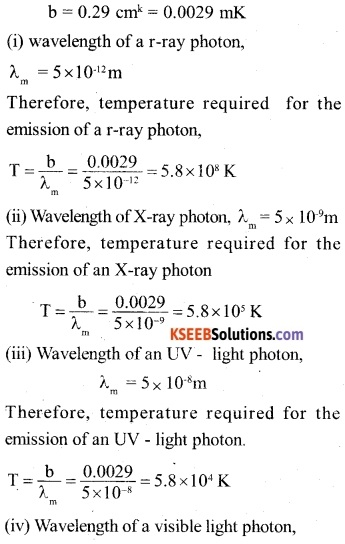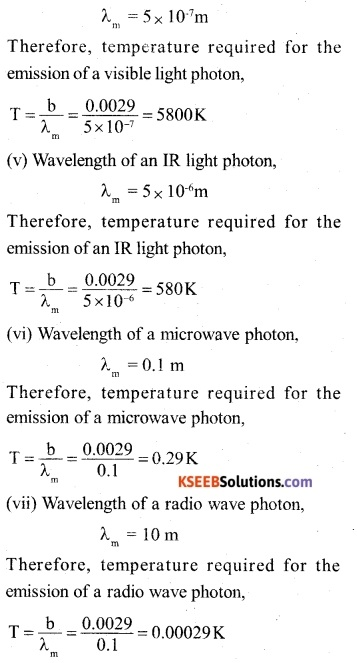These numbers tell us the order of temperature for obtaining radiations in different parts of the electromagnetic spectrum. At these temperatures, the radiation of the corresponding wavelength in a particular case can be emitted at a lower temperature also, but its intensity will not be maximum.

Question 14.
Given below are some famous numbers associated with electromagnetic radiations in different contexts in physics. State the part of the electromagnetic spectrum to which each belongs.
(a) 21 cm (wavelength emitted by atomic hydrogen in interstellar space).
(b) 1057 MHz (frequency of radiation arising from two close energy levels in hydrogen; known as Lamb shift).
(c) 7 K [temperature mwcirted with the isotropic radiation fHifag nil space-thought to be a reMc of the ‘Mg-baugtrigbi of the universe}.
(d) 5890 A – 5890 A (double lines of sodium}
(e) 14.4 keV {energy of a particular transition in 57Fe nucleus associated with a famous high-resolution spectroscopic method (Mossbauer spectroscopy)].
(a) Radiation of wavelength of 21 cm:- It corresponds to radio waves of short wavelength.

(b) Radiation of frequency of 1,057 MHz: It also corresponds to radio waves of short oil. wavelength [or of high frequency].

(c) Temperature of 2.7 K: As obtained in above question this temperature corresponds to the emission of microwaves.

(d) Double lines of sodium [5,890 A – 5,869 A]: These lines correspond to visible light in yellow. region.

(e) Energy of 14.4 ke V: As obtained in question above, this energy corresponds to X-rays of low energy (Or soft – X-rays),

Question 15.
(b) It is necessary to use. satellites for long-distance TV transmission. Why?
(c) Optical and radio telescopes are built . on the ground but X-ray astronomy is possible only from satellites orbiting the earth. Why?
(d) The small ozone layer on top of the stratosphere is crucial for human survival. Why?
(e) If the earth did not have an atmosphere, would its average surface temperature be higher or lower than what it is now?
(f ) Some scientists have predicted that a global nuclear war on the earth would be followed by a severe ‘nuclear winter’ with a devastating effect on life on earth. What might be the basis of this prediction?
(a) The shortwaves (wavelength less than 200m or frequencies greater than 1,500 kHz) are absorbed by the earth due to their high frequency but are effectively reflected by F­layer in the ionosphere. After reflection from the ionosphere, the short waves reach the surface of earth back only at a large distance from the transmitter. For this reason, short waves are used in long distance transmission.

(b) Television signals are not reflected by the ionoshpere. The TV signals from an earth station are reflected back to the earth by making use of artificial satellites.

(c) The earths atmosphere is transparent to visible light and radio waves but absorbs X-rays. Therefore, X-ray astronomy is possible only from the satellites orbiting the earth.

(d) The ultraviolet radiation from the sun is harmful to living cells and plants. The ozone layer absorbs ultra-violet radiation and prevents it from reaching the earth. It also keeps the earth warm by trapping infrared radiation.

(e) The infra-red radiation emitted by the earth are retained by the earth’s atmosphere due to the green house effect and this keeps the earth warm. If the earth didn’t have atmosphere, its average temperature would have been low.

(f) Scientists estimate that in case of global nuclear war, the clouds produced will cover probably whole of the sky. In that case, solar radiation would be prevented from reaching the earth and it will result in, what they call as nuclear winter on the earth.

Question 1.
What is Maxwell’s displacement current?
The displacement current is produced, when in an electric circuit (say across the plates of a charged capacitor), the electric field changes with time. Mathematically,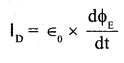Question 2.
Can an electromagnetic wave be deflected by magnetic or electric field? Explain.
No, an electromagnetic wave cannot be deflected by magnetic or electric field. It is because, they do not consist of charged particles. They merely propagate in the form of electric and magnetic fields varying both in space and time.

Question 3.
The dimensions of $$\frac { 1 }{ { \mu }_{ 0 }{ \in }_{ 0 } }$$ where symbols have their usual meanings are:
(A) [L1 T]
(B) [L2 T2]
(C) [L2 T-2]
(D) [L T1]
The velocity of the electo magnetic wave in free space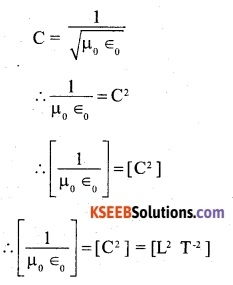Question 4.
Which of the following are not electromagnetic waves
(A) Cosmic rays
(B) γ-rays
(C) β – rays
(D) X-rays
(C) β – rays

Question 5.
If $${ \in }_{ 0 }$$ and $${ \mu }_{ 0 }$$ are the electric permeability and magnetic permeability in free space, ∈ and µ are the corresponding quantities in a medium, then index of refraction of the medium is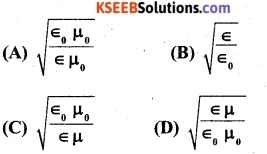The velocity of the electromagnetic waves in free space is given by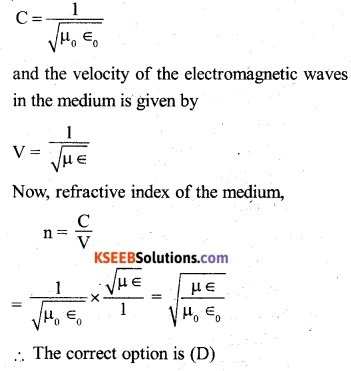Question 6.
The speed of electromagnetic waves is independent of
(A) Wavelength
(B) Frequencies
(C) Intensity
(D) Medium in which it travels
(C) IntensityQuestion 7.
If an electromagnetic wave propagating through vacuum is described by,
E = E0 sin (Kx – wt); B = B0 sin (Kx -ωt) then,
(A) E0K = Boω
(B) E0B0 = ωK
(C) E0ω=B0K
(D) E0B0=ω/K
(A) E0K = Boω

Question 8.
The oscillating magnetic field in a plane electromagnetic wave is given by
By = 8×106sin [2 × 10-6t + 300 πx] (in t) calculate the wavelength of the wave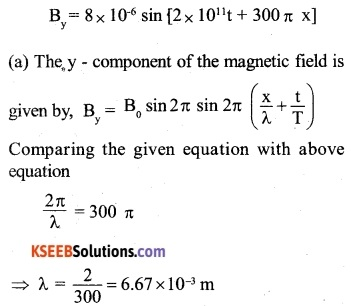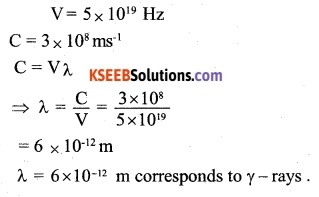The velocity of light rays of different wavelengths in a vacuum is same and hence the ratio of their velocities is 1.